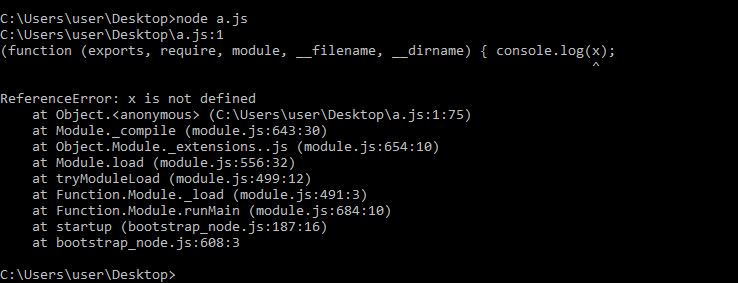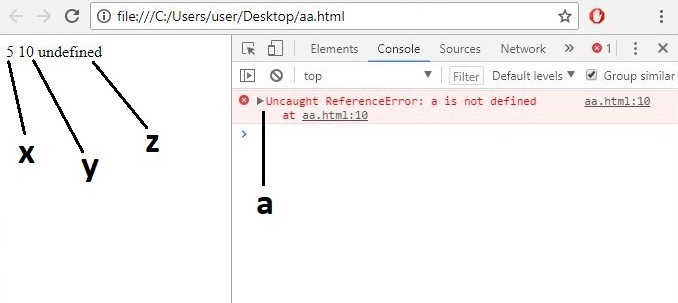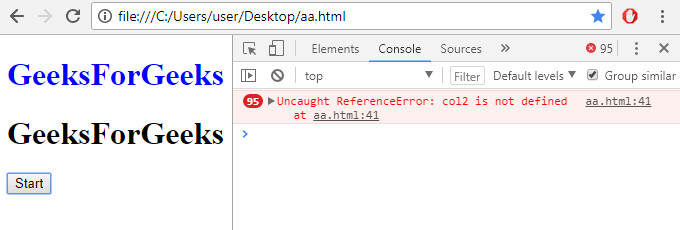# Difference between var and let in JavaScript

var and let are both used for variable declaration in javascript but the difference between them is that var is function scoped and let is block scoped.
It can be said that a variable declared with var is defined throughout the program as compared to let.
An example will clarify the difference even better
Example of var:

```Input:
console.log(x);
var x=5;
console.log(x);
Output:
undefined
5
```Example of let:

```Input:
console.log(x);
let x=5;
console.log(x);
Output:
Error
```Let’s see code in JavaScript:
Code #1:

 `<``html``> ` ` `  `<``body``> ` `    ``<``script``> ` `        ``// calling x after definition ` `        ``var x = 5; ` `        ``document.write(x, "\n"); ` ` `  `        ``// calling y after definition  ` `        ``let y = 10; ` `        ``document.write(y, "\n"); ` ` `  `        ``// calling var z before definition will return undefined ` `        ``document.write(z, "\n"); ` `        ``var z = 2; ` ` `  `        ``// calling let a before definition will give error ` `        ``document.write(a); ` `        ``let a = 3; ` `    `` ` ` ` ` `  `                             `

### OutputCode #2:
In the following code, clicking start will call a function that changes the color of the two headings every 0.5sec. The color of first heading is stored in a var and the second one is declared by using let.
Both of them are then accessed outside the function block. Var will work but the variable declared using let will show an error because let is block scoped.

 ` ` `<``html``> ` ` `  `<``head``> ` `    ``<``title``>Var vs Let ` ` ` ` `  `<``body``> ` ` `  `    ``<``h1` `id``=``"var"` `style``=``"color:black;"``>GeeksForGeeks ` `    ``<``h1` `id``=``"let"` `style``=``"color:black;"``>GeeksForGeeks ` `    ``<``button` `id``=``"btn"` `onclick``=``"colour()"``>Start ` `    ``` ` `  `    ``<``script` `type``=``"text/javascript"``> ` `        ``function colour() { ` ` `  `            ``setInterval(function() { ` ` `  `                ``if (document.getElementById('var').style.color == 'black') ` `                    ``var col1 = 'blue'; ` `                ``else ` `                    ``col1 = 'black'; ` ` `  `                ``// setting value of color 1 through var ` ` `  `                ``if (document.getElementById('let').style.color == 'black') { ` `                    ``let col2 = 'red'; ` `                ``} else { ` `                    ``col2 = 'black'; ` `                ``} ` ` `  `                ``// setting value of color 2 through let ` ` `  `                ``document.getElementById('var').style.color = col1; ` ` `  `                ``document.getElementById('let').style.color = col2; ` ` `  `                ``// changing color of h1 in html ` `            ``}, 500); ` ` `  `        ``} ` `    `` ` ` ` ` `  ` `

Output:My Personal Notes arrow_drop_upCheck out this Author's contributed articles.

If you like GeeksforGeeks and would like to contribute, you can also write an article using contribute.geeksforgeeks.org or mail your article to contribute@geeksforgeeks.org. See your article appearing on the GeeksforGeeks main page and help other Geeks.

Please Improve this article if you find anything incorrect by clicking on the "Improve Article" button below.

Article Tags :

10

Please write to us at contribute@geeksforgeeks.org to report any issue with the above content.